## A.Implication-Based Full Adder

A full adder adds three binary inputs (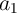,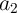, and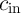) and has two outputs, sum (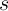) and carry (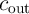) given by

 s = a1  XOR a2 XOR cin (A.1)
and
 cout = (a1 AND a2) OR (cin AND [a1 XOR a2]) ≡ (a1 AND a2) OR (cin AND [a1 OR a2]). (A.2)

In implication-based logic systems the realized logic operation is equivalent to the operation IMP or NIMP depending on the logical definitions for the high and low resistance states as explained in Table 3.2). Here the detailed implementations of a stateful full adder using the MRAM implication logic arrays are presented.

 Table A.1.: Full adder truth table.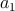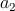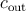0 0 0 0 0 0 0 1 1 0 0 1 0 1 0 0 1 1 0 1 1 0 0 1 0 1 0 1 0 1 1 1 0 0 1 1 1 1 1 1